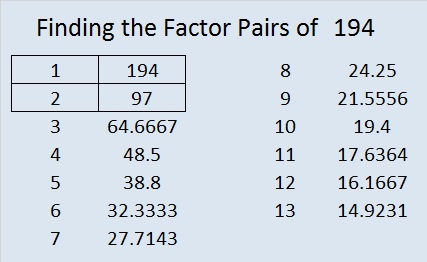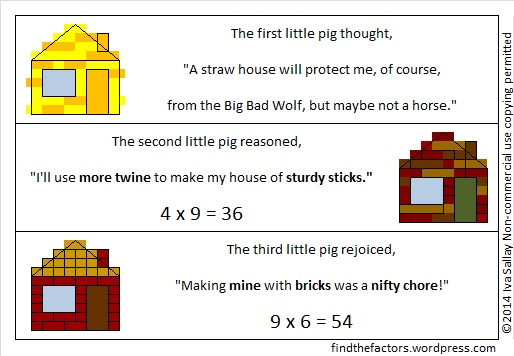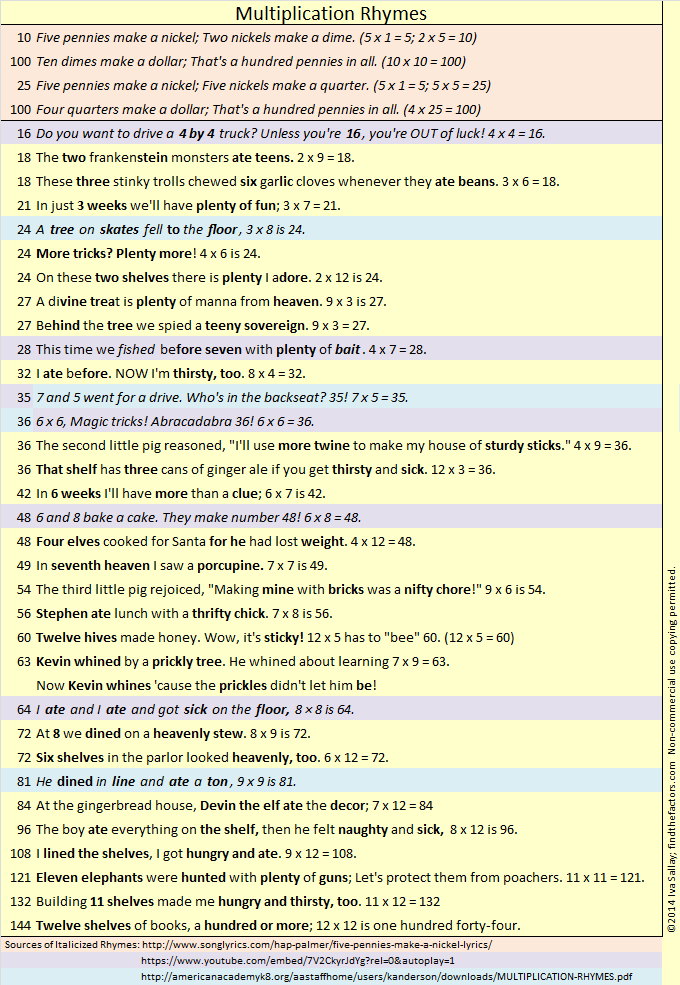# How Lucky Can 777 Be?

• 777 is a composite number.
• Prime factorization: 777 = 3 x 7 x 37
• The exponents in the prime factorization are 1, 1, and 1. Adding one to each and multiplying we get (1 + 1)(1 + 1)(1 + 1) = 2 x 2 x 2 = 8. Therefore 777 has exactly 8 factors.
• Factors of 777: 1, 3, 7, 21, 37, 111, 259, 777
• Factor pairs: 777 = 1 x 777, 3 x 259, 7 x 111, or 21 x 37
• 777 has no square factors that allow its square root to be simplified. 77727.8747197.Some people think that 7 is a lucky number. If that is true, then 777 should be even luckier.

Some numbers are lucky enough to be included in Multiplication Rhymes.ppt – mathval, a fun power point that helps students learn 12 multiplication facts, including these three that use Lucky Numbers:

• 3 & 7 are always lucky numbers; 3 x 3 = 9 lives of a cat.
• 3 & 7 are always lucky numbers; 3 x 7 = 21 lucky age.
• 3 & 7 are always lucky numbers; 7 x 7 = 49er Gold Miner.

In Number Theory Lucky Numbers are actually defined and can be generated using a sieve somewhat similar to the prime number generating Sieve of Eratosthenes. There is an infinite number of Lucky Numbers, and yes, 3, 7, 9, 21, 49, and 777 all make the list.

Oeis.org’s wiki, Lucky numbers, includes several lucky number lists including the first 33 composite Lucky Numbers thus defined because ALL of their factors are also Lucky Numbers. 777 was the 19th number on that particular list because ALL of its factors, 1, 3, 7, 21, 37, 111, 259, 777 are Lucky Numbers, too!

If that isn’t lucky enough, 777 is a repdigit in three different bases.

• 3333 BASE 6; note that 3(6^3) + 3(6^2) + 3(6^1) + 3(6^0) = 777
• 777 BASE 10; note that 7(100) + 7(10) + 7(1) = 777
• LL BASE 36 (L is 21 base 10); note that 21(36) + 21(1) = 777

Did you notice that lucky numbers 3, 7, and 21 showed  up again? I liked that coincidence so much that I made this graphic:777 is also the sum of three squares four different ways:

• 26² + 10² + 1² = 777
• 22² + 17² + 2² = 777
• 20² + 19² + 4² = 777
• 20² + 16² + 11² = 777

# 194 Are Multiplication Rhymes Able to Help Kids Learn the Multiplication Table?

194 is a composite number. Factor pairs: 194 = 1 x 194 or 2 x 97. Factors of 194: 1, 2, 97, 194. Prime factorization: 194 = 2 x 97.Are multiplication rhymes able to help kids learn the multiplication table? The obvious answer is, of course, YES! Duh!However, sometimes this obvious answer may not be correct. I recently read a researched article on the effectiveness of certain teaching practices in helping first-grade children learn mathematics. The points made in the article would likely apply to third and fourth-grade students learning multiplication as well. The article states that alternative techniques (music, movement, m&m’s, other manipulatives, etc.) do reinforce concepts for students who already understand what is being taught, but students who struggle actually make no gains when unproven techniques are used. The researcher hypothesized that when students struggle to learn mathematical concepts “alternative techniques tend to demand more, cognitively, from [these] students.” Demanding more cognitively can backfire: Requiring a student to memorize a rhyme and the math fact could put that student’s brain on overload with even fewer facts learned.

I have now modified my opinion of multiplication rhymes. If rhyme-and-rhythm is helpful, use it, if not, don’t. What is good for some of the class, may not be good for all of the class. If a student doesn’t make progress using the rhymes, it may be better to stick with more direct instruction to learn the math facts. Additionally, each rhyme should be examined individually and its own pros and cons considered.

Here are two of my FAVORITE sources of multiplication rhymes: American Academy’s MULTIPLICATION-RHYMES.pdf now requires registration to view, but it has rhymes printed in pretty colors and contains a few of my favorite multiplication rhymes. Kids would probably like looking at this pdf multiple times.

Multiplication Rhymes 1 is an entertaining You-tube video made by Mrs. Rice that children would probably enjoy watching over and over again, and it also has a few very memorable rhymes.

Although these two sources are great, I think some of the rhymes are too similar:

• From the pdf, we have “8 and 4 were sad and blue, 8 x 4 = 32” while the video recites “6 and 7 are sad and blue, they make number 42.” If students learned either of those rhymes, they may have difficulty remembering the product or which two numbers were sad and blue several weeks later.
• The video also gave two other rhymes that were too similar to each other: “9 and 8 what do they do? They go play (tag) with 72.” and  “9 and 9 are having fun. They play tag with 81.” “Tag” is in parentheses because it wasn’t on the note card that was shown, but it was spoken on the video. Again weeks later, groups or individuals may not remember which numbers were playing tag or what the product was when they were multiplied together.

I compiled my own List of Multiplication Rhymes which focus on the multiplication facts highlighted in the multiplication table below. I included some rhymes from the above pdf and the video and added some from the song Five Pennies Make a Nickel. All of those rhymes are in italics. The rhymes that I wrote myself are in regular print. If I modified an existing rhyme, the modifications are in regular print while the rest of the rhyme is in italics. I also ordered the rhymes in a way that should make finding the rhyme for any particular fact much easier. I hope there will be many rhymes on this list that you enjoy as well, and that they get used to help many students learn the very important multiplication facts highlighted in the table below.# 2 Gross Multiplication FactsThis level TWO multiplication table is only a little more difficult than the table in my previous post.  This puzzle uses 12 factors while the previous one used just 10. To find all these factors you have to be familiar with 144 multiplication facts, a requirement that sounds much more intimidating than it really is. (For example, 3 x 5 and 5 x 3 are counted as 2 different facts.) 144 is a fascinating number.  It is a dozen dozen, otherwise known as a gross. Therefore, completing this puzzle will help you review the gross multiplication facts!   If you haven’t memorized all of the facts, there is a gross way to learn some of the gross multiplication facts.

Edwin A. Anderson Elementary School uses manipulatives and rhymes to teach the multiplication facts.  Their students learn two rhymes a week for sixteen week and are masters of the gross multiplication facts when they are done. Five of the rhymes are actually a little gross:

• 3×3, nine cuts on my knee
• 3×8, 24 horseflies on my plate
• 4×7, 28 spiders are webbin’
• 6×8, fishing bait, count slimy worms all 48
• 7×7, roaches on a vine, yucky and scary all 49

It was a very gross multiplication rhyme about the number 64 that inspired me to collect some favorite rhymes and even write a few rhymes myself. I collected them in one list I titled Multiplication Rhymes:That gross, but inspiring, rhyme about the number 64 and some other gross multiplication rhymes are listed below:

• 18 The two Frankenstein monsters ate teens. 2×9=18
• 18 These three stinky trolls chewed six garlic cloves whenever they ate beans. 3×6=18
• 36 That shelf has three cans of ginger ale if you get thirsty and sick. 12×3=36
• 63 Kevin whined by a prickly tree. He whined about learning 7×9=63. Now Kevin whines ’cause the prickles didn’t let him be!
• 64 I ate and I ate and got sick on the floor, 8×8 is 64.
• 96 The boy ate everything on the shelf. Then he felt naughty and sick. 8×12 is 96.

Knowing all 144 (gross) multiplication facts will help you complete my puzzles.

As you Find the Factors and fill in those gross multiplication facts, there are two things I’d like you to remember:

1. Don’t write anything on the inside of the puzzle until you write down all the factors on the outside of the puzzle.

2. The only numbers allowed in the factor row (top row) and the factor column (1st column) are the numbers from 1 to 12, and all 12 numbers must appear in both places.

Now you can click on 12 Factors 2013-10-31, to find the 6 puzzles appearing in this post.

Now let’s think about the number 2:

2 is the smallest prime number and the only prime that is an even number.

2 is also the largest number that is equal to its number of factors.

In particular situations, there are several prefixes and words that mean two:

• 2 is the second counting number.
• A bicycle is a two-wheeler. A binomial is a polynomial with two terms.
• Two musicians form a duet. Take a look at duet’s synonyms and translations in other languages.
• The playing card with a 2 on it is called the deuce.
• A pair can be two shoes, two eyes, or lots of other possibilities.
• Two people in a relationship are called a couple.

Can you think of any more?

Base 2 uses only the digits 1 and 0 to represent each counting number. Computers use base 2, or binary, as it is also called.

Wikipedia informs us of a Calculus topic about the number 2:

• If you take the sum from 0 to ∞ of b⁻ᵏ, it will converge to b, ONLY when the base number, b, is 2. Thus,
•  ⅟₁ + ⅟₂ + ⅟₄ + ⅟₈ + ⅟₁₆ + ⅟₃₂ + ⅟₆₄ + ⅟₁₂₈ + … = 2

Here is 2’s factoring information:

• 2 is a prime number.
• Prime factorization: 2 is prime.
• The exponent of prime number 2 is 1. Adding 1 to that exponent we get (1 + 1) = 2. Therefore 2 has exactly 2 factors.
• Factors of 2: 1, 2
• Factor pairs: 2 = 1 x 2
• 2 has no square factors that allow its square root to be simplified. √2 ≈ 1.414.How do we know that 2 is a prime number? If 2 were not a prime number, then it would be divisible by at least one prime number less than or equal to √2 ≈ 1.4. Since there are no prime numbers less than or equal to 1.4, we know 2 is a prime number.

When 2 is a clue in the FIND THE FACTOR puzzles, the factors are always 1 and 2.